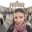Related Tags

algorithms
coding interview
c++
data structures

# How to get a random node from linked list in a single passAni Tumanyan

### Problem statement

Given a singly linked list of unknown length, return a random node from the list by only one traversal. Each node must have the same probability of being chosen.

This problem is a popular interview question. The approach discussed below is a useful technique in data streaming applications when data is too large to fit in the memory.

### Description of the algorithm

We will use the reservoir sampling technique with a reservoir size equal to 1.

The algorithm consists of the following steps:

1. Initialize the result node with the head node of the list.
2. Track number of the processed elements. After the first step $i = 1$, the head node is processed.
3. Iterate over the elements in the list:
• Generate uniformly distributed random number $r \in [0, i];$
• If the generated number $r$ equals$0$, then select the current node as a result node.
• Increment the number of processed elements: $i = i + 1;$
• Move to the next element of the list.

After these steps, the resulting node will be a random node chosen with equal probability from a given list. See the proof of correctness below:

### Complexity analysis

Let$n$ be the length of the given linked list:

• Time complexity is $O(n)$as we iterate over the whole list.
• The Space complexity is $O(1)$as constant auxiliary space is used.

### Code example

Let's look at the code below:

#include <random>

//get random number in a given range
int getRandomNumber(int left, int right)
{
//C++11's random number generation facilities
std::uniform_int_distribution<> distrib(left, right);
std::mt19937 randomGen;
return distrib(randomGen);
}

//get random node from a given linked list in a single pass
{
int i = 1;
while(currNode != nullptr)
{
int randomInd = getRandom(0, i);
if(randomInd == 0)
resultNode = currNode;
++i;
currNode = currNode->next;
}
return resultNode;
}

### Explanation

Let $n$ be the length of the given list, and prove that the probability of $i$-th node (which has an index $i-1$) to be selected as a result node is $1/n$.

• During iteration over the $i$-th node, there are already $i-1$ processed elements. We get a random number from $0$ to $i - 1$, so there are $i$ values to be chosen with equal probability.
• The probability that generated random number equals $0$ is $1/i$, so the $i$-th node is selected with probability $1/i$.
• At the end of the whole process, the $i$-th node will remain selected if it is not replaced by further nodes during iteration, which means further nodes haven't been selected.
• For $(i+1)$-th node, the probability of not being selected is $1 - 1/(i+1)$, for $(i+2)$-th node, it is, $(i+2) n$- th node $- \; 1 - 1/n$. The probability that $i$-th node remains selected after further iteration is a product of these probabilities:$(1 - \frac{1}{i+1})(1 - \frac{1}{i+2}) \dots (1 - \frac{1}{n}) = \frac{i}{n}.$
• So, the probability for $i$-th node to be selected as a result node is:

$P(i\text{-}th \; is \; selected)\cdot P(further \; nodes\;are \; not\; selected)= \frac{1}{i} \cdot \frac{i}{n}= \frac{1}{n}.$

This proves that each node is returned with equal probability $1/n$.

RELATED TAGS

algorithms
coding interview
c++
data structures

CONTRIBUTORAni Tumanyan
RELATED COURSES

View all Courses

Keep Exploring

Learn in-demand tech skills in half the time